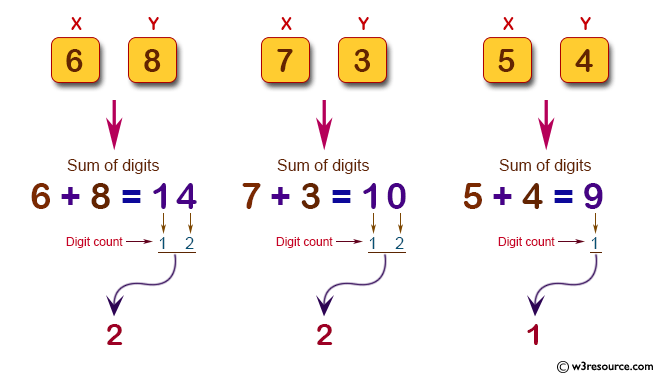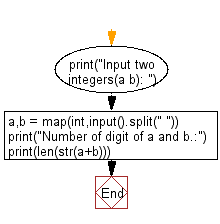﻿ Python: Compute the digit number of sum of two given integers - w3resource# Python: Compute the digit number of sum of two given integers

## Python Basic - 1: Exercise-33 with Solution

Write a Python program to compute the digit number of sum of two given integers.

Input:
Each test case consists of two non-negative integers x and y which are separated by a space in a line.
0 ≤ x, y ≤ 1,000,000

Pictorial Presentation:Sample Solution:

Python Code:

``````print("Input two integers(a b): ")
a,b = map(int,input().split(" "))
print("Number of digit of a and b.:")
print(len(str(a+b)))
``````

Sample Output:

```Input two integers(a b):
5 7
Number of digit of a and b.:
2
```

Flowchart:Python Code Editor:

Have another way to solve this solution? Contribute your code (and comments) through Disqus.

What is the difficulty level of this exercise?

Test your Python skills with w3resource's quiz

﻿

## Python: Tips of the Day

Merges two or more lists into a list of lists, combining elements from each of the input lists based on their positions

Example:

```def tips_merge(*args, fill_value=None):
max_length = max([len(lst) for lst in args])
result = []
for i in range(max_length):
result.append([
args[k][i] if i < len(args[k])
else fill_value for k in range(len(args))
])
return result
print(tips_merge(['x', 'y'], [1, 2], [True, False]))
print(tips_merge(['x'], [1, 2], [True, False]))
print(tips_merge(['x'], [1, 2], [True, False], fill_value = '_'))
```

Output:

```[['x', 1, True], ['y', 2, False]]
[['x', 1, True], [None, 2, False]]
[['x', 1, True], ['_', 2, False]]
```